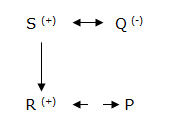# Crack IBPS PO : Seating Arrangement : Day 50

1-5) Study the following instructions carefully and answer the questions below:

A, B, C, D, K, L, M, N, S and Z are members of a family. All are like different outdoor games viz, Cricket, Baseball, Yard Yahtzee, Bon Appetit, Football, Blanket run, Lawn Twister, Giant jenga, Yard dominoes and Dunk Bucket but not necessarily in the same order. There are four married couple and three generation in the family. Football is not liked by a female. K’s brother like baseball. The one who like Blanket run is a wife of S. M is spouse of N and plays Yard Dominoes. Z is a father of K, and Z’s wife like cricket. Football and Bon Appetit is liked by one couple. S has two children. A is mother-in-law of B. S is the only son of A. B doesn’t like Blanket run. B’s father- in-law like Yard Yahtzee. M and C are cousins. All married couple have children except M and N. D is not married. K’s daughter-in-law like Dunk Bucket.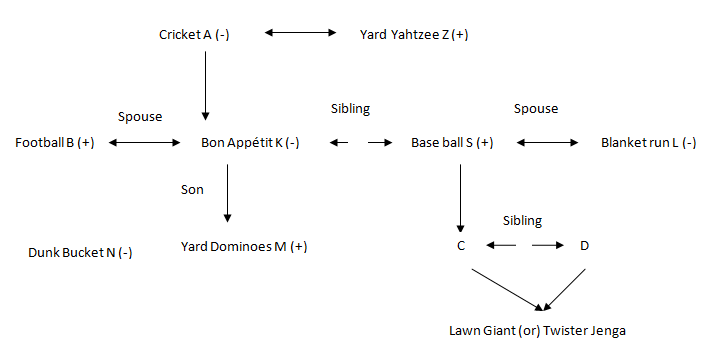1. Who is the father of the one who likes Lawn Twister?

(a) The one who like Blanket run

(b) Husband of K

(c) Son-in-law of Z

(d) The one who like baseball

(e) Cannot be determined

(d) The one who like baseball

2. Which of the following is not a married couple?

(a) K and the one who like Football

(b) A and the one who like Yard Yahtzee

(c) One who like Lawn twister and D

(d) The one who like baseball and Blanket run

(e) None of these

(c) One who like Lawn twister and D

3. Grandmother of M likes which game?

(a) Bon Appetit

(b) Blanket run

(c) Cricket

(d) Giant jenga

(e) Cannot be determined

(c) Cricket

4. Who is like Giant jenga?

(a) C

(b) M

(c) K

(d) Son of K

(e) Cannot be determined

(e) Cannot be determined

5. Who is the brother-in-law of S?

(a) N’s Father

(b) Z’s son-in-law

(c) D’s father

(d) A’s son

(e) None of these

(b) Z’s son-in-law

6. C*D means C is the mother of D; C+D means C is the brother of D; C-D means C is the wife of D; C=D means C is the daughter of D. Which of the following means N is the father of M?

(a) M+N*L-K

(b) N*M-L+K

(c) K*N-M+L

(d) K*L+M=N

(e) None of these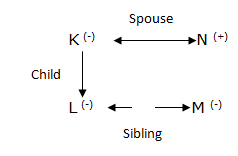7. Study the following instructions carefully and answer the questions below:

A*B’ means ‘B is the father of A’

A-B’ means ‘B is the sister of A’

A@B’ means ‘B is the brother of A’

A&B’ means ‘A is the son of B’

Which of the following indicates F is the son of D?

(a) None of these

(b) F&B*C@D

(c) F – B*C@D

(d) B@C@F&D

(e) D@A*C&F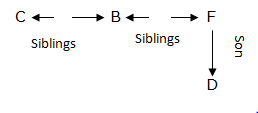8.Study the following instructions carefully and answer the questions below:

P@Q’ means ‘Q is the son of P’

P?Q’ means ‘Q is the daughter of P’

P>Q’ means ‘Q is the sister of P’

P<Q’ means ‘P is the mother of Q’

P\$Q’ means ‘Q is the father of P’

Which of the following indicates C is the sister of B?

(a) B>S, A<B, B\$D, D@C

(b) S>B, A\$D, D>C, S>A

(c) B>S, A<B, B\$D, D?C

(d) B>S, A<B, B@D, D?C

(e) None of these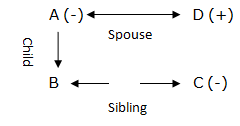9. Study the following instructions carefully and answer the questions Given below:

A*B —- A is not wife of B

A\$B —- B is the brother of A

A@B—- A is not sister of B

A%B —-A is the mother of B

A#B—-A is the son of B

S # P * Q % R \$ S

If P has one son and one daughter and T is the son in law of P.

How the son in law of Q related to S?

(a) Aunty

(b) Brother in law

(c) Uncle

(d) Can’t be determined

(e) None of these

Son-in-law of Q is T, so T is related to S as brother-in-law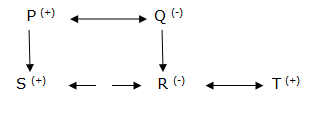10. Study the information carefully and answer the questions below:

A*B —- A is not wife of B

A\$B —- B is the brother of A

A@B—- A is not sister of B

A%B —-A is the mother of B

A#B—-A is the son of B

R is the brother of P. R who is the son of S .Q is the mother of P

Fill in the blanks with suitable symbols which will satisfy the above statement.

Q_P_R_S

(a) * @ #

(b) % \$ *

(c) \$ # %

(d) % \$ #

(e) None of these